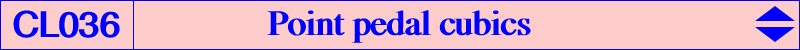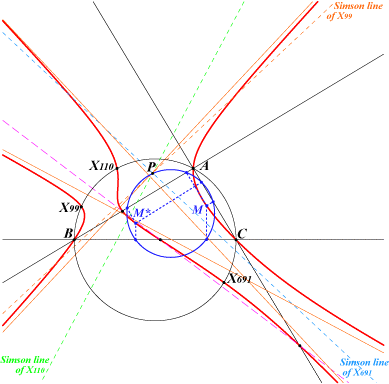Let P = p : q : r be a fixed finite point. The locus of point M whose pedal circle passes through P is in general an isogonal nK (remember that M and its isogonal conjugate M* share the same pedal circle). Its root R is the orthocorrespondent of P hence, the curve contains the orthotraces of P. This cubic is called P-pedal cubic. This cubic can never be a circular cubic nor a nK0. It is a K60 if and only if P = X(5), the nine point center and then it is K098. The H-pedal cubic is K081, an equal power cubic. A P-pedal cubic is a K+ if and only if P lies on the bicircular quartic Q032. When P lies on the nine point circle, the cubic decomposes into the rectangular circum-hyperbola with center P and the line which is its isogonal transform. The P-pedal cubic contains the given point X (and its isogonal conjugate X*) if and only if P lies on the pedal circle of X (and X*). In particular, when X lies on the circumcircle, P must lie on the Simson line of X. As a consequence, the P-pedal cubic which contains two given points Xa, Xb on the circumcircle must pass through a third point Xc also on the circumcircle: Xc is the isogonal conjugate of the infinite point of the line XaXb. Obviously, the cubic contains the isogonal conjugates Xa*, Xb*, Xc* at infinity.For example, the P-pedal cubic through X(99), X(110) contains X(691) on the circumcircle and X(512), X(523), X(690) at infinity. See figure. P is the intersection of the three Simson lines of X(99), X(110), X(691). The three asymptotes are parallel to the sidelines of the triangle formed by these three points.